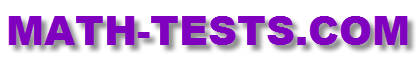#Do you want to take a 3rd grade math test to assess your math knowledge for this grade level? The following online quizzes and tests are based on the third grade math standards. Check them out!

These online tests are designed to work on computers, laptops, iPads, and other tablets. There is no need to download any app for these activities.

Use models and arrays to multiply numbers and solve multiplication problems as repeated addition.

Check your division skills and your ability to solve division word problems.

When taking this test, students will identify place value of whole numbers through thousands and round numbers to the nearest ten or hundred.

This is an interesting online test about fractions that 3rd grade students could take at the end of the chapter.

Addition and Multiplication Connection Math Test
Identify repeated addition statement equal to multiplication expression.

Division Fill in the Blank up to 10 Math Test
Solve division problems up to 10 by filling in the blank with the correct number.

Multiplication and Division Connection Math Test
Use factors to determine the correct number that makes the division statement true.

Balance Addition Equations up to 3 Digits Math Test
Identify the number (up to 3 digits) that balances the addition equation.

Write Time in Digits Math Test
Convert time in words to digital format.

Return from the 3rd Grade Math Test Page to the Math Tests homepage.